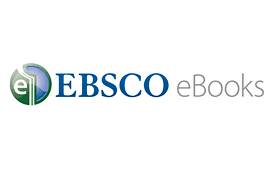# Mathematics

Check out resources on mathematics from SUNY Adirondack Library!

## Find Books

Limit to Circulating Books to find print books to borrow.

• Our library has about 30,000 print books.

• Our library has about 200,000 electronic books.

## Need help?Emily Goodspeed
Contact:
Queensbury, NY 12804
(518) 743-2200 ext. 2351
goodspeede@sunyacc.edu

## EBSCOhost eBook Collection## Mathematics Call Numbers

SUNY Adirondack Library uses The Library of Congress Call Number Classifications system to organize it's books. Most books on mathematics will be located in the QA section in the library's circulating book collection.

## Suggested Search Terms

Math* (includes Mathematics, Mathematical, Mathematicians)

Statistic* (includes Statistics, Statistical, Statisticians)

Algebra

Algorithms

Geometry

Calculus

Functions

Trigonometry

Probabilities

Logic

"Decision making"

"Number theory"

"Differential equations"